x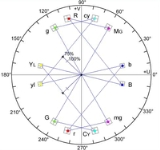Encyclopedia
Quadrature amplitude modulation (QAM) (icon or ˈ or simply "Q-A-M") is both an analog and a digital modulation
Modulation
In electronics and telecommunications, modulation is the process of varying one or more properties of a high-frequency periodic waveform, called the carrier signal, with a modulating signal which typically contains information to be transmitted...

scheme. It conveys two analog message signals, or two digital bit streams, by changing (modulating) the amplitude
Amplitude
Amplitude is the magnitude of change in the oscillating variable with each oscillation within an oscillating system. For example, sound waves in air are oscillations in atmospheric pressure and their amplitudes are proportional to the change in pressure during one oscillation...

s of two carrier wave
Carrier wave
In telecommunications, a carrier wave or carrier is a waveform that is modulated with an input signal for the purpose of conveying information. This carrier wave is usually a much higher frequency than the input signal...

s, using the amplitude-shift keying
Amplitude-shift keying
Amplitude-shift keying is a form of modulation that represents digital data as variations in the amplitude of a carrier wave.Any digital modulation scheme uses a finite number of distinct signals to represent digital data. ASK uses a finite number of amplitudes, each assigned a unique pattern of...

(ASK) digital modulation scheme or amplitude modulation
Amplitude modulation
Amplitude modulation is a technique used in electronic communication, most commonly for transmitting information via a radio carrier wave. AM works by varying the strength of the transmitted signal in relation to the information being sent...

(AM) analog modulation scheme. The two carrier waves, usually sinusoids, are out of phase with each other by 90°
Degree (angle)
A degree , usually denoted by ° , is a measurement of plane angle, representing 1⁄360 of a full rotation; one degree is equivalent to π/180 radians...

and are thus called quadrature carriers or quadrature components — hence the name of the scheme. The modulated waves are summed, and the resulting waveform is a combination of both phase-shift keying
Phase-shift keying
Phase-shift keying is a digital modulation scheme that conveys data by changing, or modulating, the phase of a reference signal ....

(PSK) and amplitude-shift keying
Amplitude-shift keying
Amplitude-shift keying is a form of modulation that represents digital data as variations in the amplitude of a carrier wave.Any digital modulation scheme uses a finite number of distinct signals to represent digital data. ASK uses a finite number of amplitudes, each assigned a unique pattern of...

(ASK), or (in the analog case) of phase modulation (PM) and amplitude modulation. In the digital QAM case, a finite number of at least two phases and at least two amplitudes are used. PSK modulators are often designed using the QAM principle, but are not considered as QAM since the amplitude of the modulated carrier signal is constant. QAM is used extensively as a modulation scheme for digital telecommunication
Telecommunication
Telecommunication is the transmission of information over significant distances to communicate. In earlier times, telecommunications involved the use of visual signals, such as beacons, smoke signals, semaphore telegraphs, signal flags, and optical heliographs, or audio messages via coded...

systems. Spectral efficiencies
Spectral efficiency
Spectral efficiency, spectrum efficiency or bandwidth efficiency refers to the information rate that can be transmitted over a given bandwidth in a specific communication system...

of 6 bits/s/Hz can be achieved with QAM.

QAM modulation is being used in optical fiber systems as bit rates increase – QAM16 and QAM64 can be optically emulated with a 3-path interferometer.

## Digital QAM

Like all modulation
Modulation
In electronics and telecommunications, modulation is the process of varying one or more properties of a high-frequency periodic waveform, called the carrier signal, with a modulating signal which typically contains information to be transmitted...

schemes, QAM conveys data
Data
The term data refers to qualitative or quantitative attributes of a variable or set of variables. Data are typically the results of measurements and can be the basis of graphs, images, or observations of a set of variables. Data are often viewed as the lowest level of abstraction from which...

by changing some aspect of a carrier signal, or the carrier wave
Carrier wave
In telecommunications, a carrier wave or carrier is a waveform that is modulated with an input signal for the purpose of conveying information. This carrier wave is usually a much higher frequency than the input signal...

, (usually a sinusoid
Sine wave
The sine wave or sinusoid is a mathematical function that describes a smooth repetitive oscillation. It occurs often in pure mathematics, as well as physics, signal processing, electrical engineering and many other fields...

) in response to a data signal. In the case of QAM, the amplitude of two waves, 90 degrees out-of-phase with each other (in quadrature) are changed (modulated or keyed) to represent the data signal. Amplitude modulating two carriers in quadrature can be equivalently viewed as both amplitude modulating and phase modulating a single carrier.

Phase modulation
Phase modulation
Phase modulation is a form of modulation that represents information as variations in the instantaneous phase of a carrier wave.Unlike its more popular counterpart, frequency modulation , PM is not very widely used for radio transmissions...

(analog PM) and phase-shift keying
Phase-shift keying
Phase-shift keying is a digital modulation scheme that conveys data by changing, or modulating, the phase of a reference signal ....

(digital PSK) can be regarded as a special case of QAM, where the magnitude of the modulating signal is a constant, with only the phase varying. This can also be extended to frequency modulation
Frequency modulation
In telecommunications and signal processing, frequency modulation conveys information over a carrier wave by varying its instantaneous frequency. This contrasts with amplitude modulation, in which the amplitude of the carrier is varied while its frequency remains constant...

(FM) and frequency-shift keying
Frequency-shift keying
Frequency-shift keying is a frequency modulation scheme in which digital information is transmitted through discrete frequency changes of a carrier wave. The simplest FSK is binary FSK . BFSK uses a pair of discrete frequencies to transmit binary information. With this scheme, the "1" is called...

(FSK), for these can be regarded as a special case of phase modulation.

## Analog QAM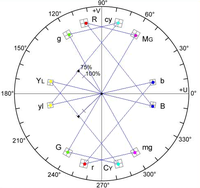When transmitting two signals by modulating them with QAM, the transmitted signal will be of the form:,

whereandare the modulating signals and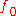is the carrier frequency.

At the receiver, these two modulating signals can be demodulated using a coherent demodulator
Product detector
A product detector is a type of demodulator used for AM and SSB signals. Rather than converting the envelope of the signal into the decoded waveform like an envelope detector, the product detector takes the product of the modulated signal and a local oscillator, hence the name...

. Such a receiver multiplies the received signal separately with both a cosine and sine
Sine
In mathematics, the sine function is a function of an angle. In a right triangle, sine gives the ratio of the length of the side opposite to an angle to the length of the hypotenuse.Sine is usually listed first amongst the trigonometric functions....

signal to produce the received estimates of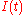and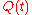respectively. Because of the orthogonality property of the carrier signals, it is possible to detect the modulating signals independently.

In the ideal caseis demodulated by multiplying the transmitted signal with a cosine signal: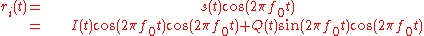Using standard trigonometric identities, we can write it as: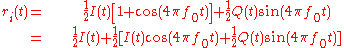Low-pass filter
Low-pass filter
A low-pass filter is an electronic filter that passes low-frequency signals but attenuates signals with frequencies higher than the cutoff frequency. The actual amount of attenuation for each frequency varies from filter to filter. It is sometimes called a high-cut filter, or treble cut filter...

ing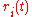removes the high frequency terms (containing), leaving only theterm. This filtered signal is unaffected by, showing that the in-phase component can be received independently of the quadrature component. Similarly, we may multiplyby a sine wave and then low-pass filter to extract.

The phase of the received signal is assumed to be known accurately at the receiver. If the demodulating phase is even a little off, it results in crosstalk between the modulated signals. This issue of carrier synchronization at the receiver must be handled somehow in QAM systems. The coherent demodulator needs to be exactly in phase with the received signal, or otherwise the modulated signals cannot be independently received. For example analog television
Analog television
Analog television is the analog transmission that involves the broadcasting of encoded analog audio and analog video signal: one in which the message conveyed by the broadcast signal is a function of deliberate variations in the amplitude and/or frequency of the signal...

systems transmit a burst of the transmitting colour subcarrier after each horizontal synchronization pulse for reference.

Analog QAM is used in NTSC
NTSC
NTSC, named for the National Television System Committee, is the analog television system that is used in most of North America, most of South America , Burma, South Korea, Taiwan, Japan, the Philippines, and some Pacific island nations and territories .Most countries using the NTSC standard, as...

and PAL
PAL
PAL, short for Phase Alternating Line, is an analogue television colour encoding system used in broadcast television systems in many countries. Other common analogue television systems are NTSC and SECAM. This page primarily discusses the PAL colour encoding system...

television systems, where the I- and Q-signals carry the components of chroma (colour) information. "Compatible QAM" or C-QUAM
C-QUAM
C-QUAM is the method of AM stereo broadcasting used in Canada, the United States and most other countries. It was invented in 1977 by Norman Parker, Francis Hilbert, and Yoshio Sakaie, and published in an IEEE journal....

is used in AM stereo
AM stereo
AM stereo is a term given to a series of mutually incompatible techniques for wireless radio broadcasting stereo audio in the AM band in a manner that is compatible with standard AM receivers...

radio to carry the stereo difference information.

### Fourier analysis of QAM

In the frequency domain
Frequency domain
In electronics, control systems engineering, and statistics, frequency domain is a term used to describe the domain for analysis of mathematical functions or signals with respect to frequency, rather than time....

, QAM has a similar spectral pattern to DSB-SC modulation. Using the properties of the Fourier transform, we find that: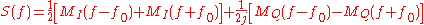where S(f), MI(f) and MQ(f) are the Fourier transforms (frequency-domain representations) of s(t), I(t) and Q(t), respectively.

## Quantized QAM

Like many digital modulation schemes, the constellation diagram
Constellation diagram
A constellation diagram is a representation of a signal modulated by a digital modulation scheme such as quadrature amplitude modulation or phase-shift keying. It displays the signal as a two-dimensional scatter diagram in the complex plane at symbol sampling instants...

is a useful representation. In QAM, the constellation points are usually arranged in a square grid with equal vertical and horizontal spacing, although other configurations are possible (e.g. Cross-QAM). Since in digital telecommunications the data are usually binary
Binary numeral system
The binary numeral system, or base-2 number system, represents numeric values using two symbols, 0 and 1. More specifically, the usual base-2 system is a positional notation with a radix of 2...

, the number of points in the grid is usually a power of 2 (2, 4, 8 ...). Since QAM is usually square, some of these are rare—the most common forms are 16-QAM, 64-QAM and 256-QAM. By moving to a higher-order constellation, it is possible to transmit more bit
Bit
A bit is the basic unit of information in computing and telecommunications; it is the amount of information stored by a digital device or other physical system that exists in one of two possible distinct states...

s per symbol. However, if the mean energy of the constellation is to remain the same (by way of making a fair comparison), the points must be closer together and are thus more susceptible to noise
Noise
In common use, the word noise means any unwanted sound. In both analog and digital electronics, noise is random unwanted perturbation to a wanted signal; it is called noise as a generalisation of the acoustic noise heard when listening to a weak radio transmission with significant electrical noise...

and other corruption; this results in a higher bit error rate and so higher-order QAM can deliver more data less reliably than lower-order QAM, for constant mean constellation energy.

If data-rates beyond those offered by 8-PSK
Phase-shift keying
Phase-shift keying is a digital modulation scheme that conveys data by changing, or modulating, the phase of a reference signal ....

are required, it is more usual to move to QAM since it achieves a greater distance between adjacent points in the I-Q plane by distributing the points more evenly. The complicating factor is that the points are no longer all the same amplitude and so the demodulator must now correctly detect both phase
Phase (waves)
Phase in waves is the fraction of a wave cycle which has elapsed relative to an arbitrary point.-Formula:The phase of an oscillation or wave refers to a sinusoidal function such as the following:...

and amplitude
Amplitude
Amplitude is the magnitude of change in the oscillating variable with each oscillation within an oscillating system. For example, sound waves in air are oscillations in atmospheric pressure and their amplitudes are proportional to the change in pressure during one oscillation...

, rather than just phase.

64-QAM and 256-QAM are often used in digital cable
Digital cable
Digital cable is a generic term for any type of cable television distribution using digital video compression or distribution. The technology was originally developed by Motorola.-Background:...

television and cable modem
Cable modem
A cable modem is a type of network bridge and modem that provides bi-directional data communication via radio frequency channels on a HFC and RFoG infrastructure. Cable modems are primarily used to deliver broadband Internet access in the form of cable Internet, taking advantage of the high...

applications. In the United States, 64-QAM and 256-QAM are the mandated modulation schemes for digital cable
Digital cable
Digital cable is a generic term for any type of cable television distribution using digital video compression or distribution. The technology was originally developed by Motorola.-Background:...

(see QAM tuner
QAM tuner
QAM stands for quadrature amplitude modulation, the format by which digital cable channels are encoded and transmitted via cable television providers...

) as standardised by the SCTE
SCTE
The Society of Cable Telecommunications Engineers or SCTE is a non-profit professional association for the advancement of technology related to cable telecommunications engineering. Founded in 1969, SCTE has a current membership of over 12,000 individuals.- Publications :SCTE offers several...

in the standard ANSI/SCTE 07 2000. Note that many marketing people will refer to these as QAM-64 and QAM-256. In the UK, 16-QAM and 64-QAM are currently used for digital terrestrial television
Digital terrestrial television
Digital terrestrial television is the technological evolution of broadcast television and advance from analog television, which broadcasts land-based signals...

(Freeview and Top Up TV
Top Up TV
Top Up TV is a pay television service in the UK launched in March 2004, operating on the digital terrestrial platform. The aim of the service is to "Top Up" Freeview customers by providing additional channels and services....

Communication systems designed to achieve very high levels of spectral efficiency
Spectral efficiency
Spectral efficiency, spectrum efficiency or bandwidth efficiency refers to the information rate that can be transmitted over a given bandwidth in a specific communication system...

usually employ very dense QAM constellations. One example is the ITU-T
ITU-T
The ITU Telecommunication Standardization Sector is one of the three sectors of the International Telecommunication Union ; it coordinates standards for telecommunications....

G.hn
G.hn
G.hn is the common name for a home network technology family of standards developed under the International Telecommunication Union's Standardization arm and promoted by the HomeGrid Forum...

standard for networking over existing home wiring (coaxial cable
Ethernet over coax
Ethernet over Coax is a family of technologies that supports the transmission of Ethernet frames over coaxial cable.- History :The first Ethernet standard, known as 10BASE5 in the family of IEEE 802.3, specified baseband operation over coaxial cable...

, phone lines and power lines
Power line communication
Power line communication or power line carrier , also known as power line digital subscriber line , mains communication, power line telecom , power line networking , or broadband over power lines are systems for carrying data on a conductor also used for electric power transmission.A wide range...

), which employs constellations up to 4096-QAM (12 bits/symbol). Another example is VDSL2 technology for copper twisted pairs, whose constellation size goes up to 32768 points.

#### Transmitter

The following picture shows the ideal structure of a QAM transmitter, with a carrier frequency
Carrier wave
In telecommunications, a carrier wave or carrier is a waveform that is modulated with an input signal for the purpose of conveying information. This carrier wave is usually a much higher frequency than the input signal...and the frequency response of the transmitter's filter: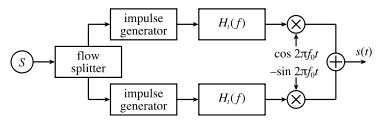First the flow of bits to be transmitted is split into two equal parts: this process generates two independent signals to be transmitted. They are encoded separately just like they were in an amplitude-shift keying
Amplitude-shift keying
Amplitude-shift keying is a form of modulation that represents digital data as variations in the amplitude of a carrier wave.Any digital modulation scheme uses a finite number of distinct signals to represent digital data. ASK uses a finite number of amplitudes, each assigned a unique pattern of...

(ASK) modulator. Then one channel (the one "in phase") is multiplied by a cosine, while the other channel (in "quadrature") is multiplied by a sine. This way there is a phase of 90° between them. They are simply added one to the other and sent through the real channel.

The sent signal can be expressed in the form:where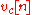andare the voltages applied in response to theth symbol to the cosine and sine waves respectively.

The receiver simply performs the inverse process of the transmitter. Its ideal structure is shown in the picture below withthe receive filter's frequency response :Multiplying by a cosine (or a sine) and by a low-pass filter it is possible to extract the component in phase (or in quadrature). Then there is only an ASK
Amplitude-shift keying
Amplitude-shift keying is a form of modulation that represents digital data as variations in the amplitude of a carrier wave.Any digital modulation scheme uses a finite number of distinct signals to represent digital data. ASK uses a finite number of amplitudes, each assigned a unique pattern of...

demodulator and the two flows of data are merged back.

In practice, there is an unknown phase delay between the transmitter and receiver that must be compensated by synchronization of the receivers local oscillator, i.e. the sine and cosine functions in the above figure. In mobile applications, there will often be an offset in the relative frequency as well, due to the possible presence of a Doppler shift proportional to the relative velocity of the transmitter and receiver. Both the phase and frequency variations introduced by the channel must be compensated by properly tuning the sine and cosine components, which requires a phase reference, and is typically accomplished using a Phase-Locked Loop (PLL)
Phase-locked loop
A phase-locked loop or phase lock loop is a control system that generates an output signal whose phase is related to the phase of an input "reference" signal. It is an electronic circuit consisting of a variable frequency oscillator and a phase detector...

.

In any application, the low-pass filter will be within hr (t): here it was shown just to be clearer.

## Quantized QAM performance

The following definitions are needed in determining error rates:
•= Number of symbols in modulation constellation
•= Energy-per-bit
Bit
A bit is the basic unit of information in computing and telecommunications; it is the amount of information stored by a digital device or other physical system that exists in one of two possible distinct states...

•= Energy-per-symbol =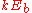with k bits per symbol
•= Noise power spectral density (W
Watt
The watt is a derived unit of power in the International System of Units , named after the Scottish engineer James Watt . The unit, defined as one joule per second, measures the rate of energy conversion.-Definition:...

/Hz
Hertz
The hertz is the SI unit of frequency defined as the number of cycles per second of a periodic phenomenon. One of its most common uses is the description of the sine wave, particularly those used in radio and audio applications....

)
•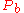= Probability
Probability
Probability is ordinarily used to describe an attitude of mind towards some proposition of whose truth we arenot certain. The proposition of interest is usually of the form "Will a specific event occur?" The attitude of mind is of the form "How certain are we that the event will occur?" The...

of bit-error
•= Probability of bit-error per carrier
•= Probability of symbol-error
•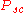= Probability of symbol-error per carrier
•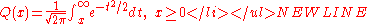.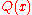is related to the complementary Gaussian error function
Error function
In mathematics, the error function is a special function of sigmoid shape which occurs in probability, statistics and partial differential equations...

by: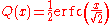, which is the probability that x will be under the tail of the Gaussian PDF
Probability density function
In probability theory, a probability density function , or density of a continuous random variable is a function that describes the relative likelihood for this random variable to occur at a given point. The probability for the random variable to fall within a particular region is given by the...

towards positive infinity.

The error rates quoted here are those in additive
Additive white Gaussian noise is a channel model in which the only impairment to communication is a linear addition of wideband or white noise with a constant spectral density and a Gaussian distribution of amplitude. The model does not account for fading, frequency selectivity, interference,...

white
White noise
White noise is a random signal with a flat power spectral density. In other words, the signal contains equal power within a fixed bandwidth at any center frequency...

Gaussian noise
Gaussian noise
Gaussian noise is statistical noise that has its probability density function equal to that of the normal distribution, which is also known as the Gaussian distribution. In other words, the values that the noise can take on are Gaussian-distributed. A special case is white Gaussian noise, in which...

(AWGN).

Where coordinates for constellation points are given in this article, note that they represent a non-normalised constellation. That is, if a particular mean average energy were required (e.g. unit average energy), the constellation would need to be linearly scaled.

### Rectangular QAM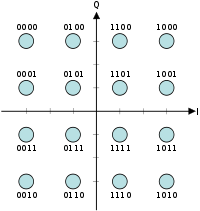Rectangular QAM constellations are, in general, sub-optimal in the sense that they do not maximally space the constellation points for a given energy. However, they have the considerable advantage that they may be easily transmitted as two pulse amplitude modulation (PAM) signals on quadrature carriers, and can be easily demodulated. The non-square constellations, dealt with below, achieve marginally better bit-error rate (BER) but are harder to modulate and demodulate.

The first rectangular QAM constellation usually encountered is 16-QAM, the constellation diagram for which is shown here. A Gray code
Gray code
The reflected binary code, also known as Gray code after Frank Gray, is a binary numeral system where two successive values differ in only one bit. It is a non-weighted code....

d bit-assignment is also given. The reason that 16-QAM is usually the first is that a brief consideration reveals that 2-QAM and 4-QAM are in fact binary phase-shift keying (BPSK) and quadrature phase-shift keying (QPSK), respectively. Also, the error-rate performance of 8-QAM is close to that of 16-QAM (only about 0.5 dB
Decibel
The decibel is a logarithmic unit that indicates the ratio of a physical quantity relative to a specified or implied reference level. A ratio in decibels is ten times the logarithm to base 10 of the ratio of two power quantities...

better), but its data rate is only three-quarters that of 16-QAM.

Expressions for the symbol-error rate of rectangular QAM are not hard to derive but yield rather unpleasant expressions. For an even number of bits per symbol,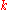, exact expressions are available. They are most easily expressed in a per carrier sense:,
so.

The bit-error rate depends on the bit to symbol mapping, but forand a Gray-coded assignment—so that we can assume each symbol error causes only one bit error—the bit-error rate is approximately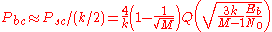.
Since the carriers are independent, the overall bit error rate is the same as the per-carrier error rate, just like BPSK and QPSK.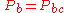.

#### Odd-k QAM

For odd, such as 8-QAM (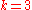) it is harder to obtain symbol-error rates, but a tight upper bound is: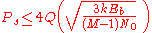.
Two rectangular 8-QAM constellations are shown below without bit assignments. These both have the same minimum distance between symbol points, and thus the same symbol-error rate (to a first approximation).

The exact bit-error rate,will depend on the bit-assignment.

Note that both of these constellations are seldom used in practice, as the non-rectangular version of 8-QAM is optimal. Example of second constellation's usage: LDPC and 8-QAM.

### Non-rectangular QAM

It is the nature of QAM that most orders of constellations can be constructed in many different ways and it is neither possible nor instructive to cover them all here. This article instead presents two, lower-order constellations.

Two diagrams of circular QAM constellation are shown, for 8-QAM and 16-QAM. The circular 8-QAM constellation is known to be the optimal 8-QAM constellation in the sense of requiring the least mean power for a given minimum Euclidean distance. The 16-QAM constellation is suboptimal although the optimal one may be constructed along the same lines as the 8-QAM constellation. The circular constellation highlights the relationship between QAM and PSK
Phase-shift keying
Phase-shift keying is a digital modulation scheme that conveys data by changing, or modulating, the phase of a reference signal ....

. Other orders of constellation may be constructed along similar (or very different) lines. It is consequently hard to establish expressions for the error rates of non-rectangular QAM since it necessarily depends on the constellation. Nevertheless, an obvious upper bound to the rate is related to the minimum Euclidean distance
Euclidean distance
In mathematics, the Euclidean distance or Euclidean metric is the "ordinary" distance between two points that one would measure with a ruler, and is given by the Pythagorean formula. By using this formula as distance, Euclidean space becomes a metric space...

of the constellation (the shortest straight-line distance between two points):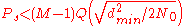.

Again, the bit-error rate will depend on the assignment of bits to symbols.

Although, in general, there is a non-rectangular constellation that is optimal for a particular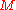, they are not often used since the rectangular QAMs are much easier to modulate and demodulate.

## Interference and noise

In moving to a higher order QAM constellation (higher data rate and mode) in hostile RF
Radio frequency is a rate of oscillation in the range of about 3 kHz to 300 GHz, which corresponds to the frequency of radio waves, and the alternating currents which carry radio signals...

/microwave
Microwave
Microwaves, a subset of radio waves, have wavelengths ranging from as long as one meter to as short as one millimeter, or equivalently, with frequencies between 300 MHz and 300 GHz. This broad definition includes both UHF and EHF , and various sources use different boundaries...

QAM application environments, such as in broadcasting
Broadcasting is the distribution of audio and video content to a dispersed audience via any audio visual medium. Receiving parties may include the general public or a relatively large subset of thereof...

or telecommunications, multipath interference
Multipath interference
Multipath interference is a phenomenon in the physics of waves whereby a wave from a source travels to a detector via two or more paths and, under the right condition, the two components of the wave interfere...

typically increases. There is a spreading of the spots in the constellation, decreasing the separation between adjacent states, making it difficult for the receiver to decode the signal appropriately. In other words, there is reduced noise immunity . There are several test parameter measurements which help determine an optimal QAM mode for a specific operating environment. The following three are most significant:
• Carrier
Carrier wave
In telecommunications, a carrier wave or carrier is a waveform that is modulated with an input signal for the purpose of conveying information. This carrier wave is usually a much higher frequency than the input signal...

/interference ratio
• Carrier-to-noise ratio
Carrier-to-noise ratio
In telecommunications, the carrier-to-noise ratio, often written CNR or C/N, is the signal-to-noise ratio of a modulated signal. The term is used to distinguish the CNR of the radio frequency passband signal from the SNR of an analogue base band message signal after demodulation, for example an...

• Threshold-to-noise ratio

• Modulation
Modulation
In electronics and telecommunications, modulation is the process of varying one or more properties of a high-frequency periodic waveform, called the carrier signal, with a modulating signal which typically contains information to be transmitted...

for other examples of modulation techniques
• Phase-shift keying
Phase-shift keying
Phase-shift keying is a digital modulation scheme that conveys data by changing, or modulating, the phase of a reference signal ....

• Amplitude and phase-shift keying
Amplitude and phase-shift keying
Amplitude and Phase-shift keying or Asymmetric Phase-shift keying, ', is a digital modulation scheme that conveys data by changing, or modulating, both the amplitude and the phase of a reference signal . In other words, it combines both Amplitude-shift keying and Phase-shift keying to increase...

or Asymmetric phase-shift keying (APSK)
• Carrierless Amplitude Phase Modulation
Carrierless Amplitude Phase Modulation
Carrierless amplitude phase modulation is a variant of quadrature amplitude modulation . Instead of modulating the amplitude of two carrier waves, CAP generates QAM signal by combining two PAM signals filtered through two filters designed so that their impulse responses form a Hilbert pair.CAP...

(CAP)
• Random modulation
Random modulation
In the theories of modulation and of stochastic processes, random modulation is the creation of a new signal from two other signals by the process of quadrature amplitude modulation. In particular, the two signals are considered as being random processes...

• QAM tuner
QAM tuner
QAM stands for quadrature amplitude modulation, the format by which digital cable channels are encoded and transmitted via cable television providers...

for HDTV